# Solution for submission 132114

A detailed solution for submission 132114 submitted for challenge IIT-M RL-ASSIGNMENT-2-TAXI

# What is the notebook about?¶

## Problem - Taxi Environment Algorithms¶

This problem deals with a taxi environment and stochastic actions. The tasks you have to do are:

• Implement Policy Iteration
• Implement Modified Policy Iteration
• Implement Value Iteration
• Implement Gauss Seidel Value Iteration
• Visualize the results
• Explain the results

## How to use this notebook? 📝¶

• This is a shared template and any edits you make here will not be saved.You should make a copy in your own drive. Click the "File" menu (top-left), then "Save a Copy in Drive". You will be working in your copy however you like.

• Update the config parameters. You can define the common variables here

Variable Description
AICROWD_DATASET_PATH Path to the file containing test data. This should be an absolute path.
AICROWD_RESULTS_DIR Path to write the output to.
AICROWD_ASSETS_DIR In case your notebook needs additional files (like model weights, etc.,), you can add them to a directory and specify the path to the directory here (please specify relative path). The contents of this directory will be sent to AIcrowd for evaluation.
AICROWD_API_KEY In order to submit your code to AIcrowd, you need to provide your account's API key. This key is available at https://www.aicrowd.com/participants/me

# Setup AIcrowd Utilities 🛠¶

We use this to bundle the files for submission and create a submission on AIcrowd. Do not edit this block.

In :
!pip install aicrowd-cli > /dev/null

ERROR: google-colab 1.0.0 has requirement requests~=2.23.0, but you'll have requests 2.25.1 which is incompatible.
ERROR: datascience 0.10.6 has requirement folium==0.2.1, but you'll have folium 0.8.3 which is incompatible.


# AIcrowd Runtime Configuration 🧷¶

Get login API key from https://www.aicrowd.com/participants/me

In :
import os

AICROWD_DATASET_PATH = os.getenv("DATASET_PATH", os.getcwd()+"/13d77bb0-b325-4e95-a03b-833eb6694acd_a2_taxi_inputs.zip")
AICROWD_RESULTS_DIR = os.getenv("OUTPUTS_DIR", "results")

In :


API Key valid
Saved API Key successfully!
13d77bb0-b325-4e95-a03b-833eb6694acd_a2_taxi_inputs.zip: 100% 31.2k/31.2k [00:00<00:00, 383kB/s]

In :
!unzip $AICROWD_DATASET_PATH  Archive: /content/13d77bb0-b325-4e95-a03b-833eb6694acd_a2_taxi_inputs.zip creating: inputs/ inflating: inputs/inputs_base.npy inflating: inputs/inputs_1.npy inflating: inputs/inputs_0.npy inflating: inputs/inputs_2.npy creating: targets/ inflating: targets/targets_2.npy inflating: targets/targets_0.npy inflating: targets/targets_1.npy inflating: targets/targets_base.npy  In : DATASET_DIR = 'inputs/'  ## Taxi Environment¶ Read the environment to understand the functions, but do not edit anything In : import numpy as np class TaxiEnv_HW2: def __init__(self, states, actions, probabilities, rewards, initial_policy): self.possible_states = states self._possible_actions = {st: ac for st, ac in zip(states, actions)} self._ride_probabilities = {st: pr for st, pr in zip(states, probabilities)} self._ride_rewards = {st: rw for st, rw in zip(states, rewards)} self.initial_policy = initial_policy self._verify() def _check_state(self, state): assert state in self.possible_states, "State %s is not a valid state" % state def _verify(self): """ Verify that data conditions are met: Number of actions matches shape of next state and actions Every probability distribution adds up to 1 """ ns = len(self.possible_states) for state in self.possible_states: ac = self._possible_actions[state] na = len(ac) rp = self._ride_probabilities[state] assert np.all(rp.shape == (na, ns)), "Probabilities shape mismatch" rr = self._ride_rewards[state] assert np.all(rr.shape == (na, ns)), "Rewards shape mismatch" assert np.allclose(rp.sum(axis=1), 1), "Probabilities don't add up to 1" def possible_actions(self, state): """ Return all possible actions from a given state """ self._check_state(state) return self._possible_actions[state] def ride_probabilities(self, state, action): """ Returns all possible ride probabilities from a state for a given action For every action a list with the returned with values in the same order as self.possible_states """ actions = self.possible_actions(state) ac_idx = actions.index(action) return self._ride_probabilities[state][ac_idx] def ride_rewards(self, state, action): actions = self.possible_actions(state) ac_idx = actions.index(action) return self._ride_rewards[state][ac_idx]  ## Example of Environment usage¶ In : def check_taxienv(): # These are the values as used in the pdf, but they may be changed during submission, so do not hardcode anything states = ['A', 'B', 'C'] actions = [['1','2','3'], ['1','2'], ['1','2','3']] probs = [np.array([[1/2, 1/4, 1/4], [1/16, 3/4, 3/16], [1/4, 1/8, 5/8]]), np.array([[1/2, 0, 1/2], [1/16, 7/8, 1/16]]), np.array([[1/4, 1/4, 1/2], [1/8, 3/4, 1/8], [3/4, 1/16, 3/16]]),] rewards = [np.array([[10, 4, 8], [ 8, 2, 4], [ 4, 6, 4]]), np.array([[14, 0, 18], [ 8, 16, 8]]), np.array([[10, 2, 8], [6, 4, 2], [4, 0, 8]]),] initial_policy = {'A': '1', 'B': '1', 'C': '1'} env = TaxiEnv_HW2(states, actions, probs, rewards, initial_policy) print("All possible states", env.possible_states) print("All possible actions from state B", env.possible_actions('B')) print("Ride probabilities from state A with action 2", env.ride_probabilities('A', '2')) print("Ride rewards from state C with action 3", env.ride_rewards('C', '3')) base_kwargs = {"states": states, "actions": actions, "probabilities": probs, "rewards": rewards, "initial_policy": initial_policy} return base_kwargs base_kwargs = check_taxienv() env = TaxiEnv_HW2(**base_kwargs)  All possible states ['A', 'B', 'C'] All possible actions from state B ['1', '2'] Ride probabilities from state A with action 2 [0.0625 0.75 0.1875] Ride rewards from state C with action 3 [4 0 8]  ## Task 1 - Policy Iteration¶ Run policy iteration on the environment and generate the policy and expected reward In : # 1.1 Policy Iteration def policy_iteration(taxienv, gamma): # A list of all the states states = taxienv.possible_states # Initial values values = {s: 0 for s in states} # This is a dictionary of states to policies -> e.g {'A': '1', 'B': '2', 'C': '1'} policy = taxienv.initial_policy.copy() ## Begin code here # Hints - # Do not hardcode anything # Only the final result is required for the results # Put any extra data in "extra_info" dictonary for any plots etc # Use the helper functions taxienv.ride_rewards, taxienv.ride_probabilities, taxienv.possible_actions # For terminating condition use the condition exactly mentioned in the pdf while True: while True: delta = 0 for s in states: j = values[s] values[s] = sum([ ( ( taxienv.ride_probabilities(s, policy[s])[states.index(sd)] ) * \ ( ( taxienv.ride_rewards(s, policy[s])[states.index(sd)] ) + (gamma * values[sd] ) ) ) for sd in states]) delta = max( delta, abs( j - values[s] ) ) if ( delta < ( 10 ** ( -8 ) ) ): break done = 1 for s in states: b = policy[s] actionValues = { currAction: sum([ ( ( taxienv.ride_probabilities(s, currAction)[states.index(sd)] ) * \ ( ( taxienv.ride_rewards(s, currAction)[states.index(sd)] ) + (gamma * values[sd] ) ) ) for sd in states]) \ for currAction in taxienv.possible_actions(s)} vals, keys = list(actionValues.values()), list(actionValues.keys()) policy[s] = keys[vals.index(max(vals))] if b != policy[s]: done = 0 if done == 1: break # Put your extra information needed for plots etc in this dictionary extra_info = {} ## Do not edit below this line # Final results return {"Expected Reward": values, "Policy": policy}, extra_info  # Task 2 - Policy Iteration for multiple values of gamma¶ Ideally this code should run as is In : # 1.2 Policy Iteration with different values of gamma def run_policy_iteration(env): gamma_values = np.arange(5, 100, 5)/100 results, extra_info = {}, {} for gamma in gamma_values: results[gamma], extra_info[gamma] = policy_iteration(env, gamma) return results, extra_info results, extra_info = run_policy_iteration(env)  # Task 3 - Modifed Policy Iteration¶ Implement modified policy iteration (where Value iteration is done for fixed m number of steps) In : # 1.3 Modified Policy Iteration def modified_policy_iteration(taxienv, gamma, m): # A list of all the states states = taxienv.possible_states # Initial values values = {s: 0 for s in states} # This is a dictionary of states to policies -> e.g {'A': '1', 'B': '2', 'C': '1'} policy = taxienv.initial_policy.copy() ## Begin code here # Hints - # Do not hardcode anything # Only the final result is required for the results # Put any extra data in "extra_info" dictonary for any plots etc # Use the helper functions taxienv.ride_rewards, taxienv.ride_probabilities, taxienv.possible_actions # For terminating condition use the condition exactly mentioned in the pdf while True: for inde in range(m+1): h = {s: 0 for s in states} for s in states: h[s] = sum([ ( ( taxienv.ride_probabilities(s, policy[s])[states.index(sd)] ) * \ ( ( taxienv.ride_rewards(s, policy[s])[states.index(sd)] ) + (gamma * values[sd] ) ) ) for sd in states]) values = h.copy() done = 1 for s in states: b = policy[s] actionValues = { currAction: sum([ ( ( taxienv.ride_probabilities(s, currAction)[states.index(sd)] ) * \ ( ( taxienv.ride_rewards(s, currAction)[states.index(sd)] ) + (gamma * values[sd] ) ) ) for sd in states]) \ for currAction in taxienv.possible_actions(s)} vals, keys = list(actionValues.values()), list(actionValues.keys()) policy[s] = keys[vals.index(max(vals))] if b != policy[s]: done = 0 if done == 1: break # Put your extra information needed for plots etc in this dictionary extra_info = {} ## Do not edit below this line # Final results return {"Expected Reward": values, "Policy": policy}, extra_info  # Task 4 Modified policy iteration for multiple values of m¶ Ideally this code should run as is In : def run_modified_policy_iteration(env): m_values = np.arange(1, 15) gamma = 0.9 results, extra_info = {}, {} for m in m_values: results[m], extra_info[m] = modified_policy_iteration(env, gamma, m) return results, extra_info results, extra_info = run_modified_policy_iteration(env)  # Task 5 Value Iteration¶ Implement value iteration and find the policy and expected rewards In : # 1.4 Value Iteration def value_iteration(taxienv, gamma): # A list of all the states states = taxienv.possible_states # Initial values values = {s: 0 for s in states} # This is a dictionary of states to policies -> e.g {'A': '1', 'B': '2', 'C': '1'} policy = taxienv.initial_policy.copy() ## Begin code here # Hints - # Do not hardcode anything # Only the final result is required for the results # Put any extra data in "extra_info" dictonary for any plots etc # Use the helper functions taxienv.ride_rewards, taxienv.ride_probabilities, taxienv.possible_actions # For terminating condition use the condition exactly mentioned in the pdf while True: delta = 0 h = {} for s in states: actionValues = { currAction: sum([ ( ( taxienv.ride_probabilities(s, currAction)[states.index(sd)] ) * \ ( ( taxienv.ride_rewards(s, currAction)[states.index(sd)] ) + (gamma * values[sd] ) ) ) for sd in states]) \ for currAction in taxienv.possible_actions(s)} vals, keys = list(actionValues.values()), list(actionValues.keys()) h[s] = max(vals) policy[s] = keys[vals.index(h[s])] delta = max( delta, abs( h[s] - values[s] ) ) values = h.copy() if ( delta < ( 10 ** ( -8 ) ) ): break # Put your extra information needed for plots etc in this dictionary extra_info = {} ## Do not edit below this line # Final results return {"Expected Reward": values, "Policy": policy}, extra_info  # Task 6 Value Iteration with multiple values of gamma¶ Ideally this code should run as is In : def run_value_iteration(env): gamma_values = np.arange(5, 100, 5)/100 results = {} results, extra_info = {}, {} for gamma in gamma_values: results[gamma], extra_info[gamma] = value_iteration(env, gamma) return results, extra_info results, extra_info = run_value_iteration(env)  # Task 7 Gauss Seidel Value Iteration¶ Implement Gauss Seidel Value Iteration In : # 1.4 Gauss Seidel Value Iteration def gauss_seidel_value_iteration(taxienv, gamma): # A list of all the states # For Gauss Seidel Value Iteration - iterate through the values in the same order states = taxienv.possible_states # Initial values values = {s: 0 for s in states} # This is a dictionary of states to policies -> e.g {'A': '1', 'B': '2', 'C': '1'} policy = taxienv.initial_policy.copy() # Hints - # Do not hardcode anything # For Gauss Seidel Value Iteration - iterate through the values in the same order as taxienv.possible_states # Only the final result is required for the results # Put any extra data in "extra_info" dictonary for any plots etc # Use the helper functions taxienv.ride_rewards, taxienv.ride_probabilities, taxienv.possible_actions # For terminating condition use the condition exactly mentioned in the pdf ## Begin code here while True: delta = 0 for s in states: j = values[s] actionValues = { currAction: sum([ ( ( taxienv.ride_probabilities(s, currAction)[states.index(sd)] ) * \ ( ( taxienv.ride_rewards(s, currAction)[states.index(sd)] ) + (gamma * values[sd] ) ) ) for sd in states]) \ for currAction in taxienv.possible_actions(s)} vals, keys = list(actionValues.values()), list(actionValues.keys()) values[s] = max(vals) policy[s] = keys[vals.index(values[s])] delta = max( delta, abs( j - values[s] ) ) if ( delta < ( 10 ** ( -8 ) ) ): break # Put your extra information needed for plots etc in this dictionary extra_info = {} ## Do not edit below this line # Final results return {"Expected Reward": values, "Policy": policy}, extra_info  # Task 8 Gauss Seidel Value Iteration with multiple values of gamma¶ Ideally this code should run as is In : def run_gauss_seidel_value_iteration(env): gamma_values = np.arange(5, 100, 5)/100 results = {} results, extra_info = {}, {} for gamma in gamma_values: results[gamma], extra_info[gamma] = gauss_seidel_value_iteration(env, gamma) return results, extra_info results, extra_info = run_gauss_seidel_value_iteration(env)  # Generate Results ✅¶ In : # Do not edit this cell def get_results(kwargs): taxienv = TaxiEnv_HW2(**kwargs) policy_iteration_results = run_policy_iteration(taxienv) modified_policy_iteration_results = run_modified_policy_iteration(taxienv) value_iteration_results = run_value_iteration(taxienv) gs_vi_results = run_gauss_seidel_value_iteration(taxienv) final_results = {} final_results["policy_iteration"] = policy_iteration_results final_results["modifed_policy_iteration"] = modified_policy_iteration_results final_results["value_iteration"] = value_iteration_results final_results["gauss_seidel_iteration"] = gs_vi_results return final_results  In : # Do not edit this cell, generate results with it as is if not os.path.exists(AICROWD_RESULTS_DIR): os.mkdir(AICROWD_RESULTS_DIR) for params_file in os.listdir(DATASET_DIR): kwargs = np.load(os.path.join(DATASET_DIR, params_file), allow_pickle=True).item() results = get_results(kwargs) idx = params_file.split('_')[-1][:-4] np.save(os.path.join(AICROWD_RESULTS_DIR, 'results_' + idx), results)  # Check your local score¶ This score is not your final score, and it doesn't use the marks weightages. This is only for your reference of how arrays are matched and with what tolerance. In : # Check your score on the given test cases (There are more private test cases not provided) target_folder = 'targets' result_folder = AICROWD_RESULTS_DIR def check_algo_match(results, targets): param_matches = [] for k in results: param_results = results[k] param_targets = targets[k] policy_match = param_results['Policy'] == param_targets['Policy'] rv = [v for k, v in param_results['Expected Reward'].items()] tv = [v for k, v in param_targets['Expected Reward'].items()] rewards_match = np.allclose(rv, tv, rtol=3) equal = rewards_match and policy_match param_matches.append(equal) return np.mean(param_matches) def check_score(target_folder, result_folder): match = [] for out_file in os.listdir(result_folder): res_file = os.path.join(result_folder, out_file) results = np.load(res_file, allow_pickle=True).item() idx = out_file.split('_')[-1][:-4] # Extract the file number target_file = os.path.join(target_folder, f"targets_{idx}.npy") targets = np.load(target_file, allow_pickle=True).item() algo_match = [] for k in targets: algo_results = results[k] algo_targets = targets[k] algo_match.append(check_algo_match(algo_results, algo_targets)) match.append(np.mean(algo_match)) return np.mean(match) if os.path.exists(target_folder): print("Shared data Score (normalized to 1):", check_score(target_folder, result_folder))  Shared data Score (normalized to 1): 1.0  ## Visualize results of Policy Iteration with multiple values of gamma¶ Add code to visualize the results In : import matplotlib.pyplot as plt print("Visualization of Policy Iteration Results\n") results, _ = run_policy_iteration(env) val = [sum(list(results[gamma]['Expected Reward'].values())) for gamma in list(results.keys())] plt.plot(list(results.keys()), val) plt.xlabel("Gamma") plt.ylabel("Net Value") plt.title("Variation of Policy Iteration Net Value with different values of Gamma") plt.show() aVal = [results[gamma]['Expected Reward']['A'] for gamma in list(results.keys())] plt.plot(list(results.keys()), aVal) plt.xlabel("Gamma") plt.ylabel("Value at A") plt.title("Variation of Policy Iteration Value at A with different values of Gamma") plt.show() bVal = [results[gamma]['Expected Reward']['B'] for gamma in list(results.keys())] plt.plot(list(results.keys()), bVal) plt.xlabel("Gamma") plt.ylabel("Value at B") plt.title("Variation of Policy Iteration Value at B with different values of Gamma") plt.show() cVal = [results[gamma]['Expected Reward']['C'] for gamma in list(results.keys())] plt.plot(list(results.keys()), cVal) plt.xlabel("Gamma") plt.ylabel("Value at C") plt.title("Variation of Policy Iteration Value at C with different values of Gamma") plt.show() aPol = [int(results[gamma]['Policy']['A']) for gamma in list(results.keys())] plt.plot(list(results.keys()), aPol) plt.xlabel("Gamma") plt.ylabel("Policy at A") plt.title("Variation of Policy Iteration Policy at A with different values of Gamma") plt.show() bPol = [int(results[gamma]['Policy']['B']) for gamma in list(results.keys())] plt.plot(list(results.keys()), bPol) plt.xlabel("Gamma") plt.ylabel("Policy at B") plt.title("Variation of Policy Iteration Policy at B with different values of Gamma") plt.show() cPol = [int(results[gamma]['Policy']['C']) for gamma in list(results.keys())] plt.plot(list(results.keys()), cPol) plt.xlabel("Gamma") plt.ylabel("Policy at C") plt.title("Variation of Policy Iteration Policy at C with different values of Gamma") plt.show()  Visualization of Policy Iteration Results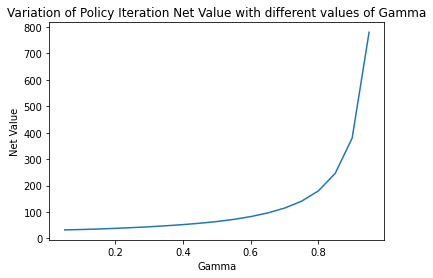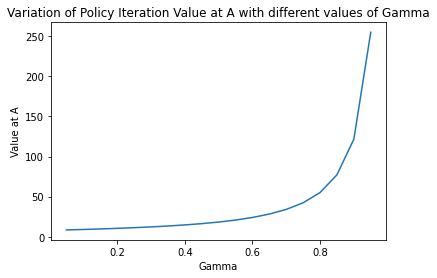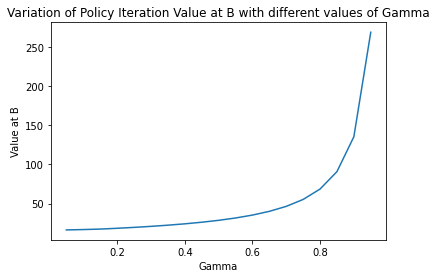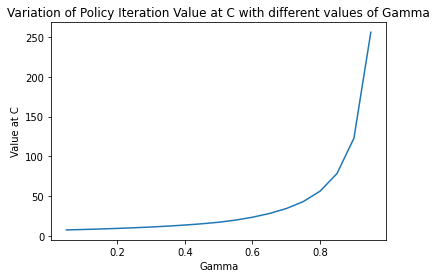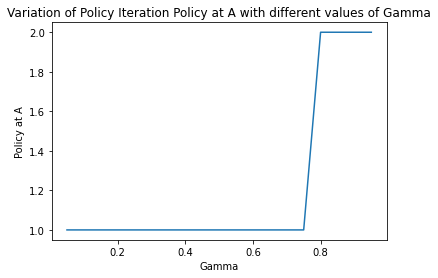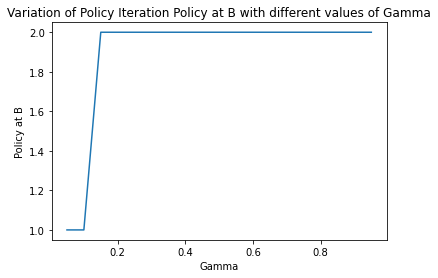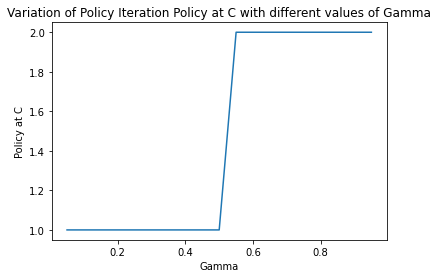In : print("Visualization of Modified Policy Iteration Results\n") results, _ = run_modified_policy_iteration(env) val = [sum(list(results[gamma]['Expected Reward'].values())) for gamma in list(results.keys())] plt.plot(list(results.keys()), val) plt.xlabel("M") plt.ylabel("Net Value") plt.title("Variation of Modified Policy Iteration Net Value with different values of M") plt.show() aVal = [results[gamma]['Expected Reward']['A'] for gamma in list(results.keys())] plt.plot(list(results.keys()), aVal) plt.xlabel("M") plt.ylabel("Value at A") plt.title("Variation of Modified Policy Iteration Value at A with different values of M") plt.show() bVal = [results[gamma]['Expected Reward']['B'] for gamma in list(results.keys())] plt.plot(list(results.keys()), bVal) plt.xlabel("M") plt.ylabel("Value at B") plt.title("Variation of Modified Policy Iteration Value at B with different values of M") plt.show() cVal = [results[gamma]['Expected Reward']['C'] for gamma in list(results.keys())] plt.plot(list(results.keys()), cVal) plt.xlabel("M") plt.ylabel("Value at C") plt.title("Variation of Modified Policy Iteration Value at C with different values of M") plt.show() aPol = [int(results[gamma]['Policy']['A']) for gamma in list(results.keys())] plt.plot(list(results.keys()), aPol) plt.xlabel("M") plt.ylabel("Policy at A") plt.title("Variation of Modified Policy Iteration Policy at A with different values of M") plt.show() bPol = [int(results[gamma]['Policy']['B']) for gamma in list(results.keys())] plt.plot(list(results.keys()), bPol) plt.xlabel("M") plt.ylabel("Policy at B") plt.title("Variation of Modified Policy Iteration Policy at B with different values of M") plt.show() cPol = [int(results[gamma]['Policy']['C']) for gamma in list(results.keys())] plt.plot(list(results.keys()), cPol) plt.xlabel("M") plt.ylabel("Policy at C") plt.title("Variation of Modified Policy Iteration Policy at C with different values of M") plt.show()  Visualization of Modified Policy Iteration Results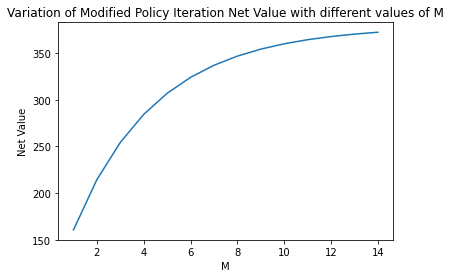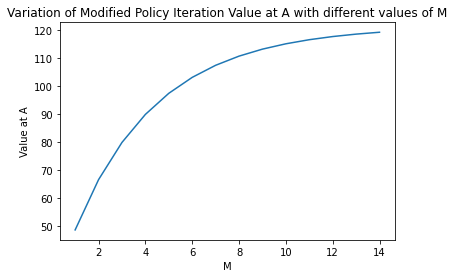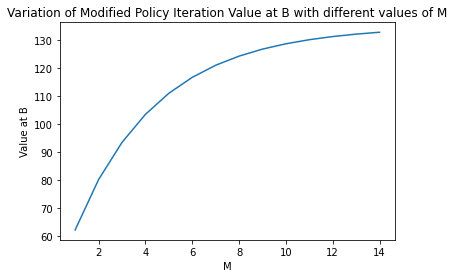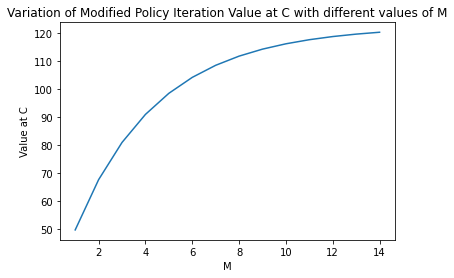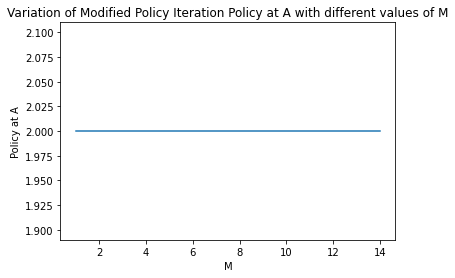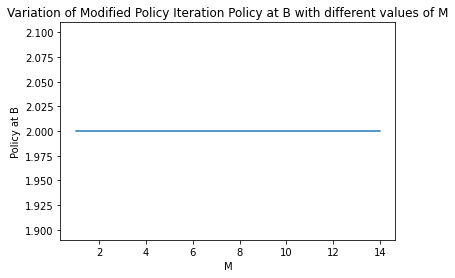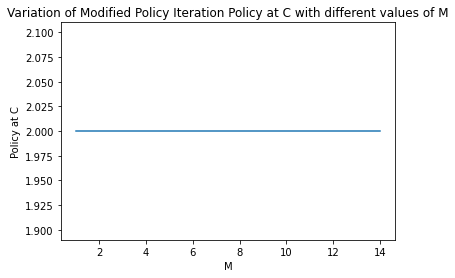# Subjective questions¶ ## 1.a How are values of$\gamma$affecting results of policy iteration¶ Higher values of gamma result in convergence to a higher value for the value each state and even the net value. Another consequence this leads to is quicker convergence to the ideal policy at each state with a higher gamma. This can be interpreted from the graphs plotted. ## 1.b For modified policy itetaration, do you find any improvement if you choose m=10.¶ With respect to the values, they don't saturate till 'm' is higher than 14. So using 'm' = 10 is obviously beneficial than using lower values of 'm' with respect to values but increasing 'm' beyond 10 doesn't lead to a significant improvement. With respect to policies, they converge to the ideal policy for much lower values of 'm' so using 'm'=10 doesn't lead to significant improvement in that respect. This can be interpreted from the graphs plotted. ## 1.c Compare and contrast the behavior of Value Iteration and Gauss Seidel Value Iteraton¶ One of the main differences between Value Iteration and Gauss Seidel Iteration is that: In Value Iteration, for performing the value updates for each state sequentially we use only the values obtained at the end of the previous iteration for computing the update. However for doing the same in Gauss Seidel we make instantaneous value updates and use the latest value of a previously computed state to make the value update for a state which comes later in the sequence within the same iteration instead of using the values obtained at the end of the previous iteration. The primary difference that this creates is that the policy in gauss seidel converges more quickly to the ideal policy than that obtained in value iteration and therefore the value also saturates to a higher level earlier in Gauss Seidel than in Value Iteration # Submit to AIcrowd 🚀¶ In [ ]: !DATASET_PATH=$AICROWD_DATASET_PATH aicrowd notebook submit -c iit-m-rl-assignment-2-taxi -a assets

WARNING: No assets directory at assets... Creating one...
No jupyter lab module found. Using jupyter notebook.
Using notebook: /content/IITM_Assignment_2_Taxi_Release.ipynb for submission...